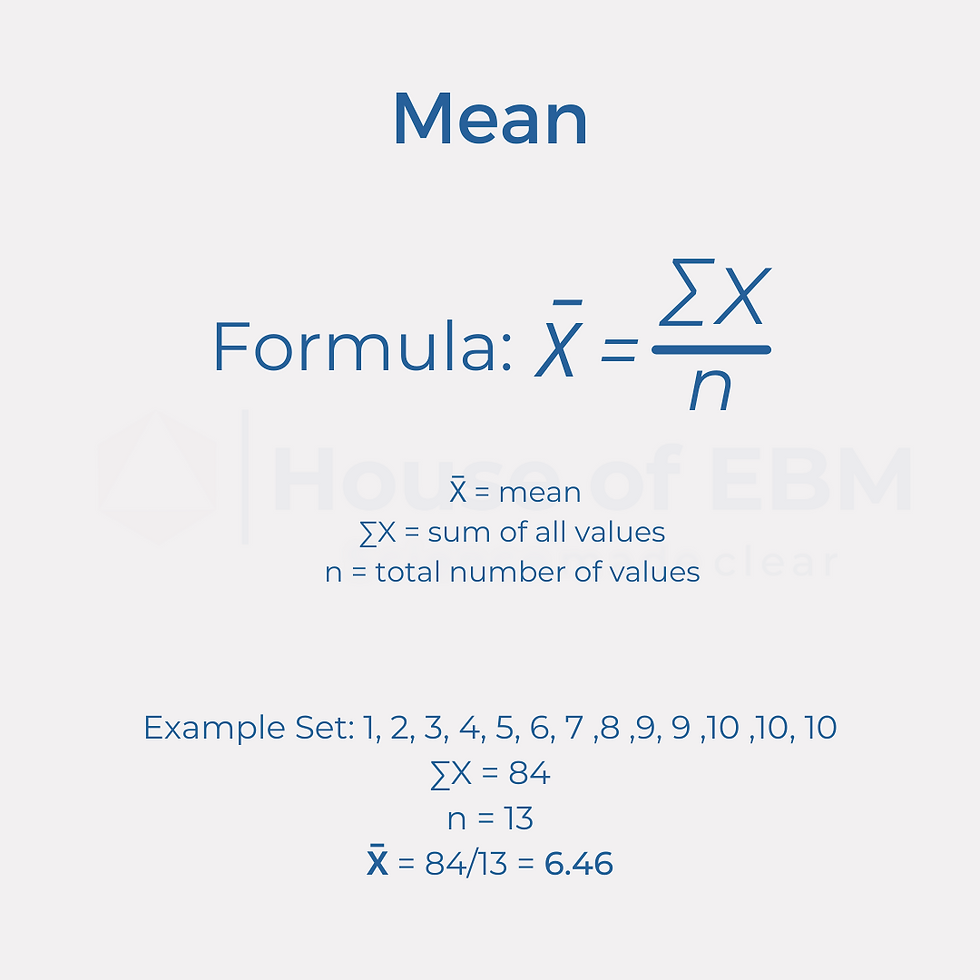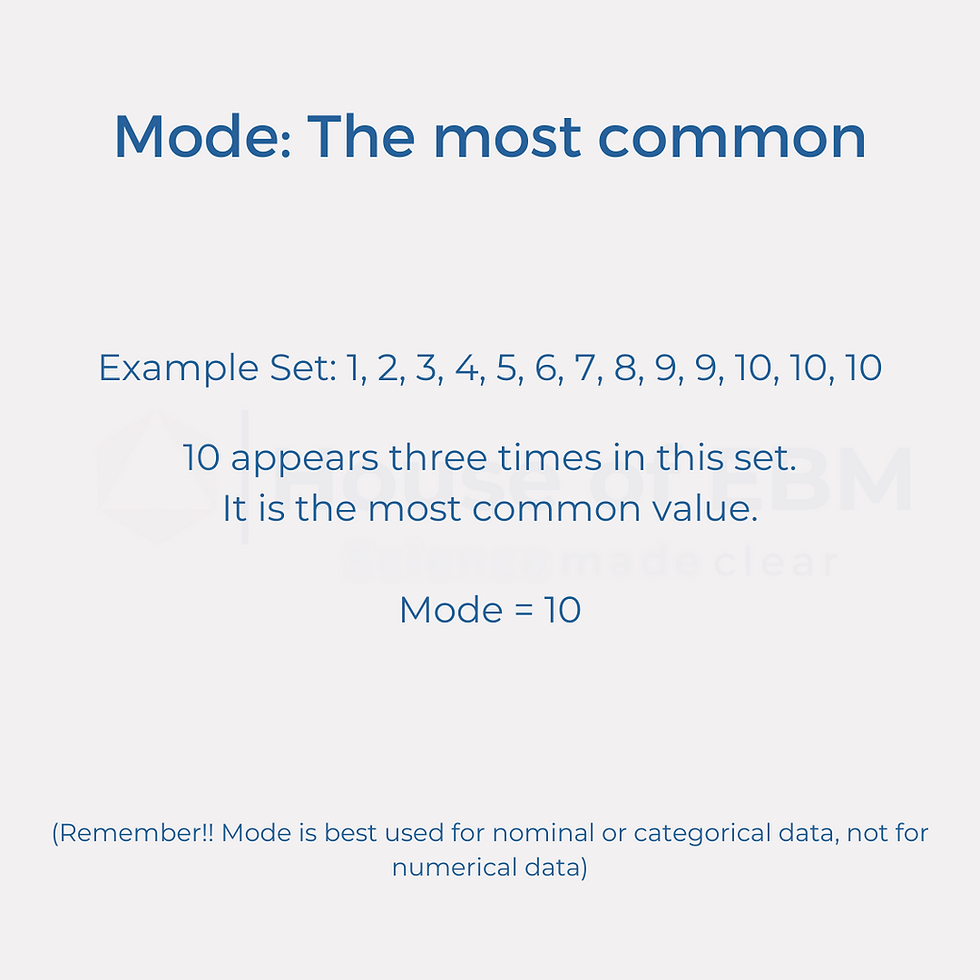top of page
Szukaj
• Agastya Patel

# Measures of Central Tendency: How to represent your data?

### What is it all about?

Measures of Central Tendency are a way to statistically identify a single value which accurately describes and is a representative of an entire data set. They are part of "descriptive" statistics.

The three most commonly used measures of central tendency are:

• Mean

• Median

• Mode

### Mean

Mean (average) is, by far, the most widely utilized central tendency measure.

Surely, you have had to calculate means in math classes during your school years. But, just to have a quick refresher, it is typically calculated as shown below:How to calculate mean? By House of EBM

There are several types of it such as arithmetic mean, weighted arithmetic mean, geometric mean and harmonic mean. The most typically used of these is arithmetic mean, and it is the one we have described above. (We discuss the other types of mean in our online courses.)

### When to use mean?

Distribution of data: Normally distributed

• If data is normally distributed, then means is the best way to describe a dataset.

• If data is not normally distributed, meaning that it is skewed, then the mean value will not accurately represent the center point of the data set, because it is more likely to be influenced and skewed towards the right or left.

Type of data: Numerical data

• Examples: Average time between symptom onset and diagnosis; Average BMI etc.

Measure of Variation: Standard deviation (SD)

Other considerations: Outliers

• Outliers are values which differ greatly from other values in a data set and are at the extremes (very low or very high).

• Outliers have significant impact on mean calculation.

• They can cause either overestimation or underestimation of the mean value.

### Median

Median describes the value which is exactly at the middle point of a data set, when it is arranged from lowest to highest.

The method of identifying the middle value of data set depends on if the total number of observations in the set is odd or even.

• For an odd number of observations, the median value lies at the (n+1)/2 position of the data set.Calculating median for odd-number dataset. By House of EBM

• For an even number of observations, the median value is the mean of the two middle values in the data set, that is the mean of values at n/2 and (n+1)/2 position.Calculating median for even-number dataset. By House of EBM

### When to use median?

Distribution of data: Non-normally distributed

• Median is a better representation for data sets which are left- or right-skewed as it is the least affected by outliers or distribution.

• It can also be used for normally distributed data, however, mean is a more accurate descriptor for such data.

Type of data: Numerical data

• Examples: Average time between symptom onset and diagnosis; Average BMI etc.

Measure of Variation: Interquartile range (IQR)

### Mode

Mode is simply the value which appears most frequently in the data set. It is identified as shown below:How to find the mode value? By House of EBM

### When to use mode?

Distribution of data: Normally distributed

Type of data: Categorical data.

• In clinical research, mode is most widely used to describe the category with the highest number of subjects.

• For example, a study has 50 males and 70 females. The mode for this category will be females, as they are the most common value.

• In such cases, mode can be easily identified either by counting the value which appears most frequently or plotting a bar graph, where the value with the highest bar will be the mode.

• It can also be used to describe numerical (interval or ratio) data.

### RecapCommon measures of central tendency. By House of EBM

EBM 101 course, where we further discuss these concepts and how to use them in research

EBM 201 course, where we show you how to use statistical software to perform such analysis for your data and how to build the "statistical analysis" section of your methods section.

Courses available in English and Polish at https://courses.houseofebm.com

Instagram: @house_of_ebm